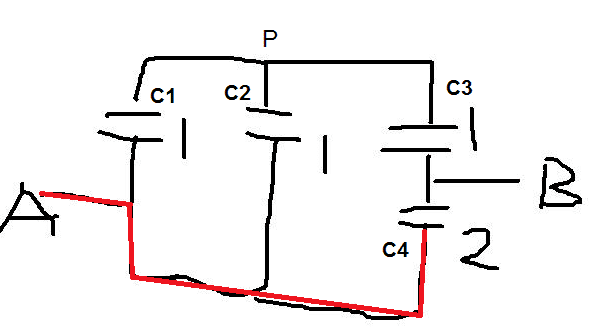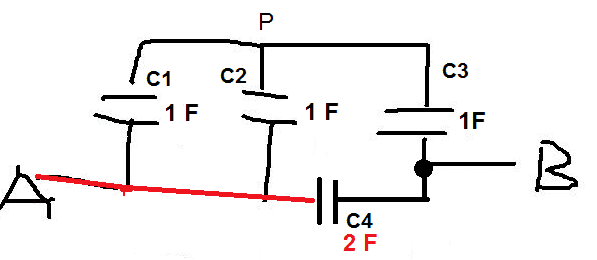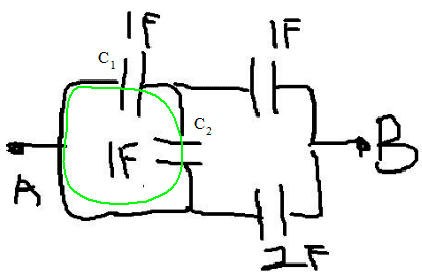# Find Equivalent Capacitance Between A & B with Capacitor

• WeiLoong
In summary, the conversation discussed the concept of parallel and series connections in a circuit and how to determine if components are connected in parallel through tracing a closed path. The example given was analyzed and it was determined that C1 and C2 are parallel and C3 and C4 are not in series. The conversation also highlighted the importance of understanding topology in determining parallel and series connections.

## Homework Statement

https://scontent-kul1-1.xx.fbcdn.net/v/t34.0-12/13228153_10206233880597531_197570509_n.png?oh=32466b5e1ab50b8c8a0f49be9d044411&oe=573C076F
Find the equivalent capacitance between A and B

Capacitor

## The Attempt at a Solution

I assume 1 and 2 is in parallel
There for 1/C = 1/1+1/1+1/3
C=8/3
Seems like this idea is not so convincing, Anyone has better idea on how to solve this question?

Parallel components are connected between same two points. Which capacitors are in parallel then?
Also, your formula for equivalent capacitance for parallel capacitors is incorrect.

@WeiLoong
I assume 1 and 2 is in parallel
There for 1/C = 1/1+1/1+1/3
C=8/3
Seems like this idea is not so convincing, Anyone has better idea on how to solve this question?

Which capacitors are 1 and 2? C1 and C2 are really parallel, but what about the others? Somehow you presented a correct result for the equivalent capacitance, but how? Where is that 1/3 from?
The red line means the same node A in the circuit.WeiLoong said:
I assume 1 and 2 is in parallel
There for 1/C = 1/1+1/1+1/3
C=8/3
there is some error in addition.
moreover your circuit given above does not show the relation.
check it.

ehild said:
@WeiLoong

Which capacitors are 1 and 2? C1 and C2 are really parallel, but what about the others? Somehow you presented a correct result for the equivalent capacitance, but how? Where is that 1/3 from?
The red line means the same node A in the circuit.

View attachment 100804
c1 and c2 is parallel meanwhile c3 and c4 are series
c3 and c4 will give me 2/3F
2/3F is parallel to C1 and C2
hence C=1+1+2/3=8/3 is that correct?

WeiLoong said:
c1 and c2 is parallel meanwhile c3 and c4 are series

No, C3 and C4 are not in series, as their common point is connected to B. How is the equivalent of C1 and C2 connected to C3?so that mean C1 C2 C3 are parrallel?
C123= 3F?

WeiLoong said:
so that mean C1 C2 C3 are parrallel?
C123= 3F?
cnh1995 said:
Parallel components are connected between same two points.

https://scontent-kul1-1.xx.fbcdn.net/v/t34.0-12/13231083_10206234376729934_1801165661_n.png?oh=6c602ace0e3669a2fc756dec529a82d2&oe=573C5142
Like this? Then 2F and 1 F on top are in series

WeiLoong said:
https://scontent-kul1-1.xx.fbcdn.net/v/t34.0-12/13231083_10206234376729934_1801165661_n.png?oh=6c602ace0e3669a2fc756dec529a82d2&oe=573C5142
Like this? Then 2F and 1 F on top are in series
Right.

WeiLoong said:
so that mean C1 C2 C3 are parrallel?
C123= 3F?
Just because components can be drawn so that their symbols are parallel does not mean that they are connected in parallel. Parallel and series connectivity is a matter of topology, not drawing aesthetics. On a schematic diagram wires and components can be moved or turned or twisted or routed or placed all over the page, but so long as the connections remain the same, the topology of the circuit remains the same.

You need to get a firm grasp on what parallel and series connections mean and be able to recognize the necessary and sufficient conditions for both.

Here is a quick test that will let you recognize if two components are in parallel: If you can trace a complete, closed path through the two components following existing wiring and without passing through any other component along the way, then those two components are parallel.

Your C1 and C2 are such a pair:Note the closed path in green that passes only through C1 and C2 and no other components along the way.

WeiLoong said:
https://scontent-kul1-1.xx.fbcdn.net/v/t34.0-12/13231083_10206234376729934_1801165661_n.png?oh=6c602ace0e3669a2fc756dec529a82d2&oe=573C5142
Like this? Then 2F and 1 F on top are in series
Yes.